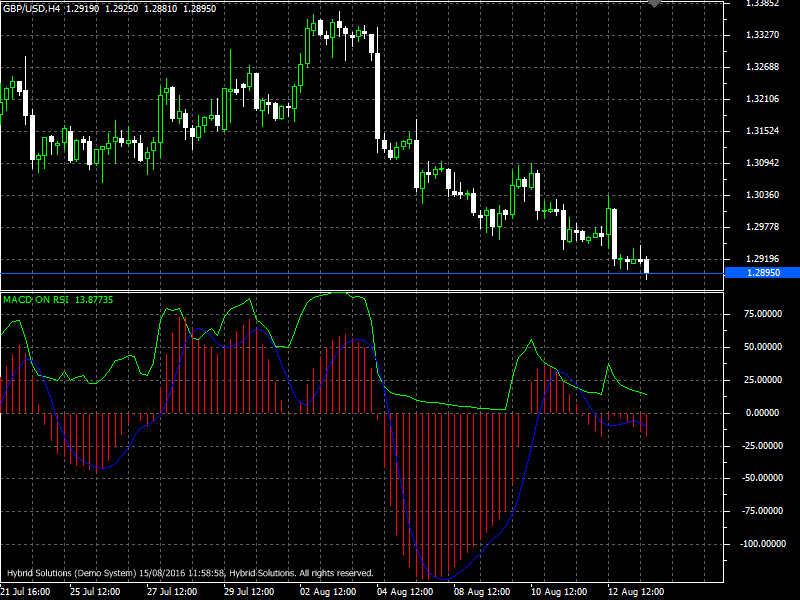# MACD on RSI

Free

In stock

MACD on RSI is a powerful VertexFX client side VTL indicator that gives precise entry and exit signals based on the MACD histogram drawn on the basis of the RSI Smoothed Oscillator.

This indicator is a combination of the MACD Crossover and the Relative Strength Index (RSI). Relative Strength Index(RSI) indicator applied to Moving Average Convergence/Divergence (MACD) clearly shows the current price divergence. And Crossover of MACD signal line and RSI is an additional signal for market entry.

SKU: 1266 Categories: ,MACD on RSI is a powerful VertexFX client side VTL indicator that gives precise entry and exit signals based on the MACD histogram drawn on the basis of the RSI Smoothed Oscillator.

This indicator is a combination of the MACD Crossover and the Relative Strength Index (RSI). Relative Strength Index(RSI) indicator applied to Moving Average Convergence/Divergence (MACD) clearly shows the current price divergence. And Crossover of MACD signal line and RSI is an additional signal for market entry.

The MACD is scaled up so that the two indicators overlap. Red line is the signal line of the histogram, green is the RSI oscillator drawn on the Moving Average values. The indicator is made so that short period reversals (1, 5, 15 min) are also clearly seen.

A buy signal is generated when the MACD crosses the signal line from the MACD histogram below the zero mark. RSI line crosses at the same level from the bottom 30 to the top.
A sell signal is generated when the MACD crosses the signal line from the MACD histogram is above the zero mark. RSI crosses this line at the level 70 downwards.